# Electronics and Communication Engineering - Electromagnetic Field Theory

36.
Calculate the directivity of a given linear end fire, uniform array of 20 element, each separated by λ/3
100
10
26.66
30
Explanation: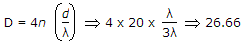.

37.
For a 400 kHz transmission line having L = 0.5 mH/km, C = 0.08 mF and negligible R and G, the velocity propagation will be
15.8303 km/s
15.830 km/s
1.583 km/s
0.1583 km/s
Explanation: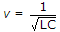m/sec.

38.
Antenna efficiency of antenna is, if radiation resistance is 370 Ω and loss resistance is 100 Ω
80%
87%
78%
21.27%
Explanation: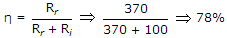.

39.
At a particular time it is observed that the frequencies of F layer at Delhi is 3 MHz the maximum electron density is
11 x 1010
1.1 x 1010
0.11 x 1010
11 x 1012
Explanation:

fc = 9Nmax3 x 106 = 9Nmax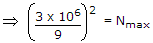Nmax = 0.11 x 101211 x 1010/m3.

40.
The effective length of an λ/2 antenna (Given Rr = 73 Ω and (Ae)max = 0.13 λ2 and η = 120 p is __________ .
0.6348 λ
0.317 λ
0.158 λ
0.371 λ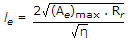.